# 2-Dimensional Shapes and Their Properties

 Listen to this Lesson:

In grade 5, students learn about the different 2-dimensional shapes, such as circles, squares, triangles, etc. They gain an in-depth understanding of what these shapes represent, and learn how to identify their properties.

Lessons on 2-dimensional shapes can be lots of fun if math teachers are equipped with the right resources! To this end, we bring you a few teaching ideas and awesome activities on this topic. Use them in your class and see students’ math knowledge soar in no time!## Ideas for Teaching 2-Dimensional Shapes and Their Properties

### What Are 2-Dimensional Shapes?

For starters, define what 2-dimensional shapes are. Explain that a 2-dimensional shape (or a 2-D shape) is a shape that only has two measurable dimensions – length and width. It is a flat plane figure that has no depth or thickness.

By now, students have come across many 2-dimensional shapes. Add that there are many different 2-dimensional shapes and draw examples of such shapes on the whiteboard. If you have manipulatives or images, even better. You can include shapes such as the following: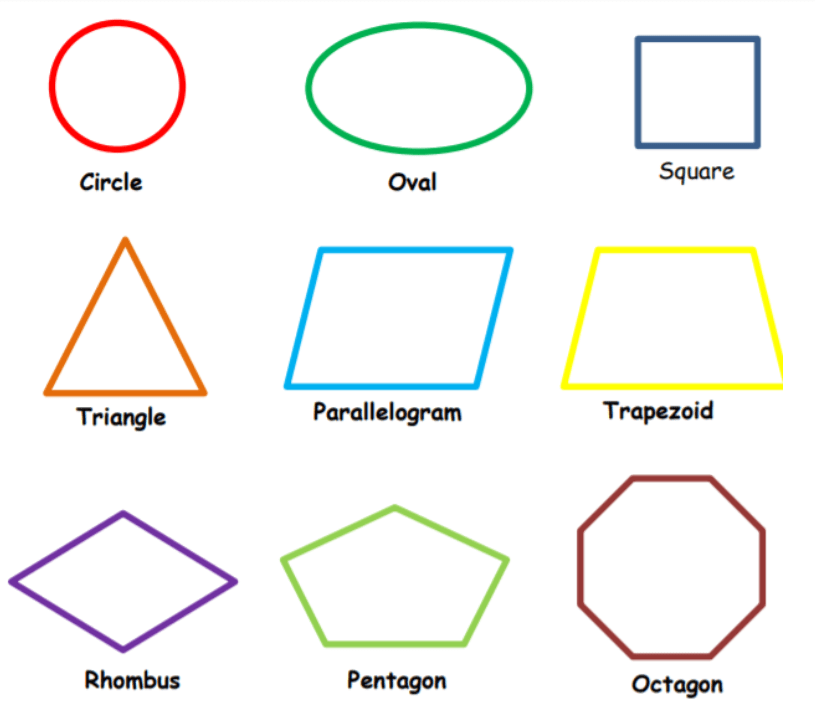Students have also encountered 3-dimensional shapes. You can draw a distinction between the two to make things clearer. While 2-dimensional shapes are flat figures with length and width, 3-dimensional shapes are solid figures with length, width, and height.

Draw an example on the whiteboard where there are both 2-dimensional figures and 3-dimensional figures and ask students to circle the 2-dimensional ones. This could look something like this: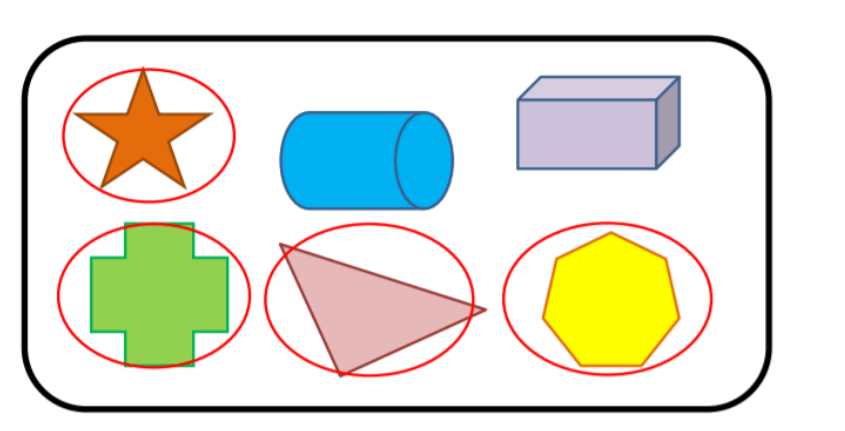If students understand the difference between 2-dimensional and 3-dimensional shapes, they should be able to recognize that the cylinder and rectangular prism have height in addition to length and width, and are not flat, hence they’re not 2-dimensional objects.

### Properties of 2-Dimensional Figures

Point out that there are many 2-dimensional figures around us and that each of them has its own unique properties. These properties make each 2-dimensional figure special, while there are also some that share common characteristics.

Add that one example of 2-D figures are polygons. Explain that a polygon is a closed plane figure, formed by connecting segments that are called ‘sides’. It’s a 2-dimensional figure whose name is based on the number of its sides. Thus, we have triangles, squares, rectangles, etc.

Ask students to reflect on the word polygon. Point out that in Greek, ‘poly’ means ‘many’, whereas ‘gon’ means ‘angle’. So polygon means ‘many angles’, and this refers to the angles formed by the straight lines (or sides) of the polygon.

Add that a polygon always has straight sides – if the figure has one side that has a curve, it does not represent a polygon. Also, polygons are always closed shapes – if the figure is open, it does not represent a polygon.

Draw a few figures on the whiteboard, and make sure to include shapes that are polygons and shapes that aren’t. Ask students to circle the ones that are polygons and explain why these qualify as polygons. Then, provide examples of polygons and their properties.

#### Example 1 – Triangle:

Draw a triangle on the whiteboard and highlight that a triangle is a 2-dimensional figure that has 3 sides that may or may not be equal. Every triangle has 3 vertices, 3 angles that may or may not be equal: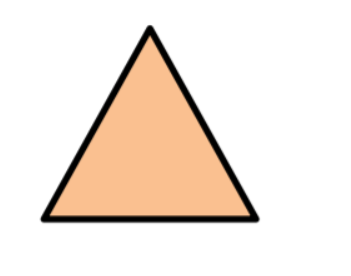Draw a quadrilateral on the whiteboard and explain that a quadrilateral is a 2-dimensional figure that has 4 sides and 4 vertices. Add that a quadrilateral is just a flat figure with four sides, all of which connect up and are straight.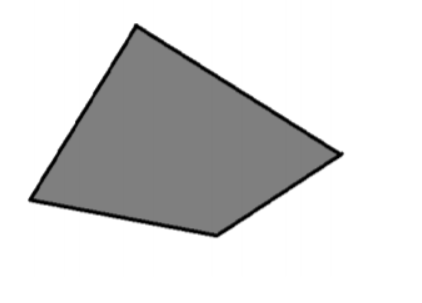#### Example 3 – Parallelogram:

Draw a parallelogram and point out that this is a quadrilateral and thus a 2-D figure. It has 4 sides, 4 vertices, 2 pairs of opposite sides that are parallel. Every parallelogram also has 2 pairs of opposite sides that are equal, as well as 2 pairs of opposite angles that are equal.#### Example 4 – Square:

Draw a square and point out that it falls into the category of quadrilaterals. Add that a square is a 2-dimensional figure that has 4 equal sides, 4 vertices, 2 pairs of parallel sides, and 4 right angles.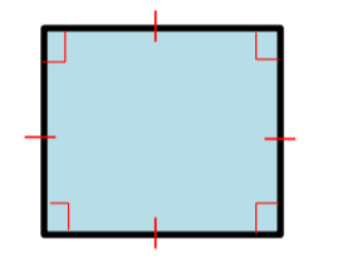#### Example 5 – Rectangle:

Draw a rectangle and add that this is a type of quadrilateral. It’s a 2-D figure that has 2 pairs of opposite sides that are congruent, as well as 4 vertices and 2 pairs of parallel sides. Every rectangle has 4 right angles.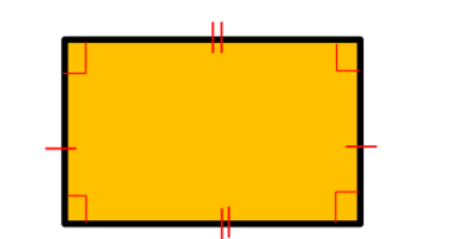#### Example 5 – Rhombus:

Draw a rhombus on the whiteboard. Add that this is a 2-dimensional figure that has 4 equal sides (a quadrilateral), 4 vertices, 2 pairs of parallel sides, and 2 pairs of opposite angles that are equal.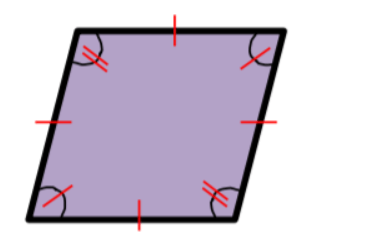#### Example 6 – Trapezoid:

Draw a trapezoid and add that this is a quadrilateral. It’s a 2-dimensional figure that has 4 sides and 4 vertices. It also has one pair of parallel sides. It can have a pair of equal sides, pairs of equal angles, as well as right angles.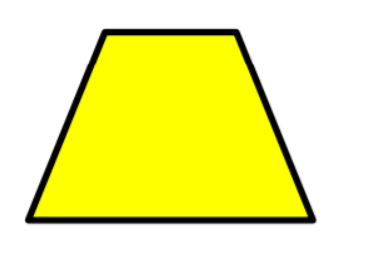#### Example 7 – Kite:

Draw a kite on the whiteboard, which also falls into the category of quadrilateral figures. Explain that a kite is a 2-dimensional figure that has 4 sides and 4 vertices. A kite has no pair of parallel sides, and it has 2 pairs of consecutive sides that are equal.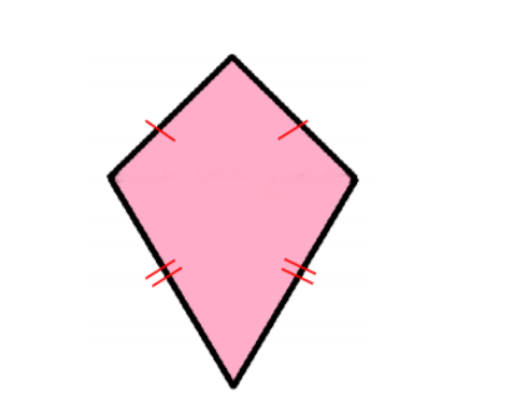#### Example 8 – Pentagon

Draw a pentagon on the whiteboard. Point out that a pentagon is a 2-dimensional figure that has 5 sides that can be equal, and also has 5 angles that can be equal.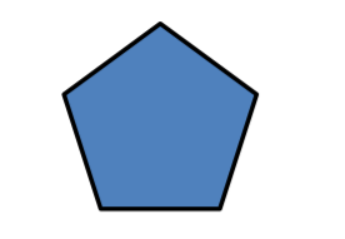#### Example 9 – Hexagon

Draw a hexagon on the whiteboard and explain that this is a 2-dimensional figure that has 6 sides that can be equal, as well as 6 angles that can be equal.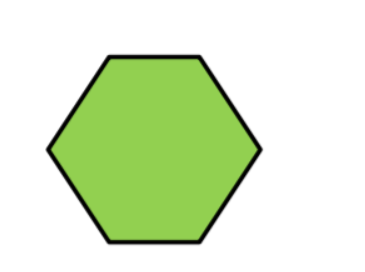#### Example 10 – Heptagon

Draw an example of a heptagon on the whiteboard. Point out that a heptagon is a 2-dimensional figure that has 7 sides that can be equal, in addition to 7 angles that can be equal.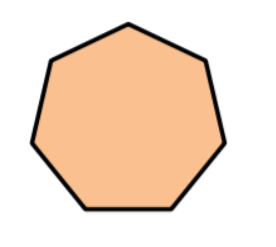#### Example 11 – Octagon

Draw an example of an octagon on the whiteboard. Explain that this is a 2-dimensional figure that has 8 sides that can be equal. It also has 8 angles that can be equal.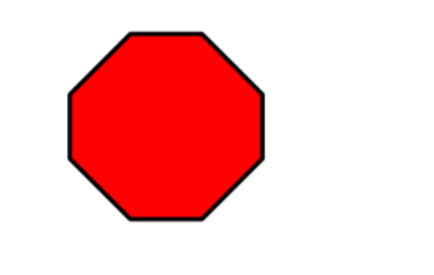### Types of Polygons

Explain that 2-dimensional figures such as polygons are classified into two categories: regular and irregular polygons. All the sides and angles or regular polygons are equal, while irregular polygons have unequal sides and angles. Draw examples of the two: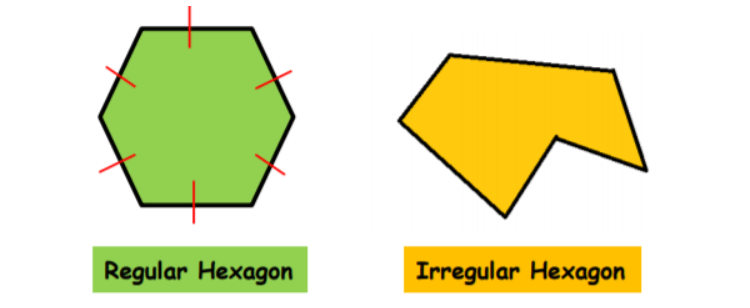Point out that in the first figure, all sides and angles are equal, so this is a regular polygon. In the second figure, on the other hand, the sides and angles are unequal, which makes the second figure an irregular polygon.

Add that there are a lot more polygons on the list, and as mentioned, each polygon is named according to the number of its sides.

#### Is a Circle a Polygon?

Draw a circle on the whiteboard and ask students to observe it and to reflect whether this figure represents a polygon.Explain that a circle is a closed plane figure. It is not a polygon because it doesn’t have corners or edges. It has a center whose distance to the edge of the circle is always the same. Add that although a circle is not a polygon, it’s still a 2-dimensional shape.

If you have the technical means in your classroom, you could also enrich your lesson on 2-dimensional shapes and their properties with multimedia material, such as videos. This is especially useful for illustrating the variety of figures.

For instance, use this video as an introduction to 2-dimensional shapes, and to the topic of what having 2 dimensions actually means. Then, play this video on recognizing the different shapes, such as circle, triangle, square, parallelogram, etc.

This video is a great resource for illustrating where the shapes exist in everyday life, which is achieved through a fun song on shapes and their properties. In addition, this video that also consists of a song called ‘Come and Meet the 2-D Shapes’ is guaranteed to make your lesson more amusing.

## Activities to Practice 2-Dimensional Shapes

### Compare Shapes Game

This is a simple online game developed by Khan Academy where students will practice their knowledge of the different 2-D shapes and their properties. To use the game as an activity in your class, make sure there are enough devices for the whole class.

Divide students into pairs and provide instructions for the game. Point out that in each exercise in the game, students have to compare two given shapes and determine which one fulfills the properties in the specific question.

In the end, students in each pair compare their results and discuss their answers. The person with the highest score in each pair wins the game. Homeschooling parents can adjust the game to an individual one.

### Properties of Shapes Game

In this brief online game by Khan Academy, students get to apply their knowledge of properties of 2-dimensional shapes. To implement the game in your class, provide suitable devices for students.

Arrange students in pairs. The two students in each pair play together and try to answer the questions correctly. In each exercise, they have to identify all answers that are true for a given statement on a 2-dimensional figure.

By doing so, the game provides a peer-tutoring approach as well. If students get stuck, they can also use a hint in the game or watch a related video for help. Parents who are homeschooling can also use the game for individual practice.

### 2-D and 3-D Shape Sort: Factory

This is a fun online game where students practice differentiating between 2-dimensional figures as flat objects with 2 dimensions and 3-dimensional figures as solid objects with 3 dimensions. The only materials needed for the game are suitable devices.

Students play the game individually. Provide instructions. Point out that in the game, students are asked to help Muggo clean up and sort his shapes into two boxes: one of which is a 2-D box and the other one a 3-D box.

Once students are done sorting the shapes into the right box, ask them to share their thoughts on the exercise. How did they know which shape goes into which box? Which dimensions do 2-D shapes have and which ones do 3-D shapes have?

In this online game on 2-D shapes, students get to use their skills at recognizing the types of quadrilaterals, as well as their properties. Provide a suitable device for each student and play the game!

Arrange students in pairs. Provide instructions for the game. Explain that in each exercise, students are given an image of a 2-dimensional figure and a few descriptions. Players have to select all descriptive words that apply to the given image.

This is a fast-paced game, so students should aim to answer as many questions as possible and as quickly as possible. In the end, the two players in each pair compare their final scores. The person with the highest score wins the game.

### ‘What Am I?’ Game

This is a fun guessing game that is bound to get students excited about 2-d shapes. To implement this game in your class, you’ll need construction paper, some scissors, and some markers.

Draw task cards on the construction paper and write a question on guessing a 2-d shape on each task card. For instance, you can create a question such as: ‘I have four sides and four angles, but my sides are never parallel. What am I?’

Include the answer under the question on each task card as well. For example, in the above case, you would include the word ‘kite’ under the question. Create as many task cards as necessary, depending on the size of your class.

Divide students into pairs and place the task cards in the middle, face down. Use at least 20 cards per pair. Provide instructions for the game. Payer 1 draws a card and reads the question to player 2. Player 2 answers the question.

If the answer is correct, they score 1 point. If the answer is incorrect, they lose 2 points. Player 2 then repeats the procedure by drawing a new card with a question for player 1. Keep playing until the pair runs out of cards.

At this moment, students calculate their final scores and decide on the winner. You can also include a symbolic prize for the person that wins the game in each pair, such as not having to do homework all week long.

## Before You Leave…

If you enjoyed these ideas and activities for teaching 2-dimensional shapes and their properties, you’ll want to check out our lesson that’s dedicated to this topic! So if you need guidance to structure your class and teach it, sign up for our emails to receive loads of free content!

Feel free to also check out our blog – you’ll find plenty of awesome resources that you can use in your class, such as this awesome article with free worksheets and activities on classifying 2-dimensional shapes!